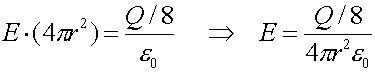Examples for Gauss's law

Example #1

Problem:

Consider a sphere of radius R=8.0m with a charge Q=3.0 mC uniformly distributed throughout the volume. What is the electric field at a distance r=4.0m?

Solution:

If one draws a Gaussian surface with radius r, the charge contained inside is one eighth of the total charge, because volume goes proportional to r3. Thus Gauss law becomes:E = 210 V/m

Example #2

Problem:Consider a point charge Q=4.0nC inside a conducting shell which has the same charge Q spread somewhere along the sphere. What are the charges on the inner/outer surface of the sphere?

Solution:

Drawing a Gaussian surface through the conductor, shows that there must be a charge of -Q on the inner surface, while the outer surface must have a charge +2Q, to make the total charge of the shell +Q.

- 4.0 nC     and   + 8.0 nC